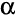# Completely Randomized Single Factor Experiment

A completely randomized single factor experiment is an experiment where both:

• One factor of two or more levels has been manipulated. For example, the experiment may be investigating the effect of different levels of price, or different flavors, or different advertisements. Where two factors are manipulated, such as both price and flavor being varied, it is then a Multifactor Experiment and not a single factor experiment.
• Each respondent in the survey is shown one and only one of the levels of the factor. For example, each respondent may be shown a single product concept, one of multiple alternative advertisements or one of multiple pricing structures. In the language of statistics, this is referred to as a completely randomized experiment.

## How to set up a completely randomized single factor experiment in Q

The most straightforward approach to analyzing a completely randomized experiment in Q is to:

1. Have the groups represented by a Pick One question.
2. Represent the outcome variable (i.e., the dependent variable) as whichever Question Type is appropriate (also see the section below on Multiple outcome variables).
3. Select each of the questions in either of the Blue or Brown Drop-down Menus (it does not matter which question is selected in which menu).
4. Select the cells on the table and press### Example

This example tests a numeric dependent variable, set up as a Number question, by three treatment groups, represented as a Pick One question. The QPack of this example is File:Completely Randomized Experiment with Numeric Outcome.QPack.

Particular aspects to note about the output are:

• An F-Test (One-Way ANOVA) has been conducted and, in this case, indicates that there is a difference between at least two of the groups.
• Multiple comparisons have also been conducted between the categories. Additionally, Column Comparisons can be added directly to the table.

See Planned ANOVA-Type Tests for more detail on the interpretation of the outputs.

## Changing test type (e.g., non-parametric tests)

In the example above a standard F-test has been conducted. Other tests can be conducted by:

See ANOVA-Type Tests - Comparing Three or More Groups for more information on how to control the test that is conducted and, more generally, see Statistical tests categorized by data type.

## Interpreting the automatic significance tests

The automatic tests of statistical significance, shown by the arrows, are not the standard significance tests typically used with an experiment. In the example above, for example, the blue font and blue arrow for Group 3 indicates that the the average value of the dependent variable is different to the combined data of the other two groups, using and Independent Samples t-Test - Comparing Two Means with Equal Variances. See Reading Tables and Interpreting Significance Tests for more information about the interpretation of the standard tests of significance.

## Multiple outcome variables

Where there are multiple outcome variables, there are three strategies for jointly testing differences:

• Where the questions containing the variables are all categorical, create a Pick Any question containing all of the variables (flattening Pick One - Multi questions if required) and then conduct the test using this new composite question.
• Where the questions containing the variables are all numeric, or, some numeric and some categorical variables, create a Number - Multi question containing all of the variables, converting any categorical variables to binary variables and flattening Pick One - Multi questions if required, and then conduct the test using this new composite question.
• Create a Tree with the outcomes selected as Questions to analyze, the group variable selected as splitting by questions and, in Advanced, the Model selection criterion as AIC.